Blog Archives

ggplot2: Faceting

April 24, 2018
By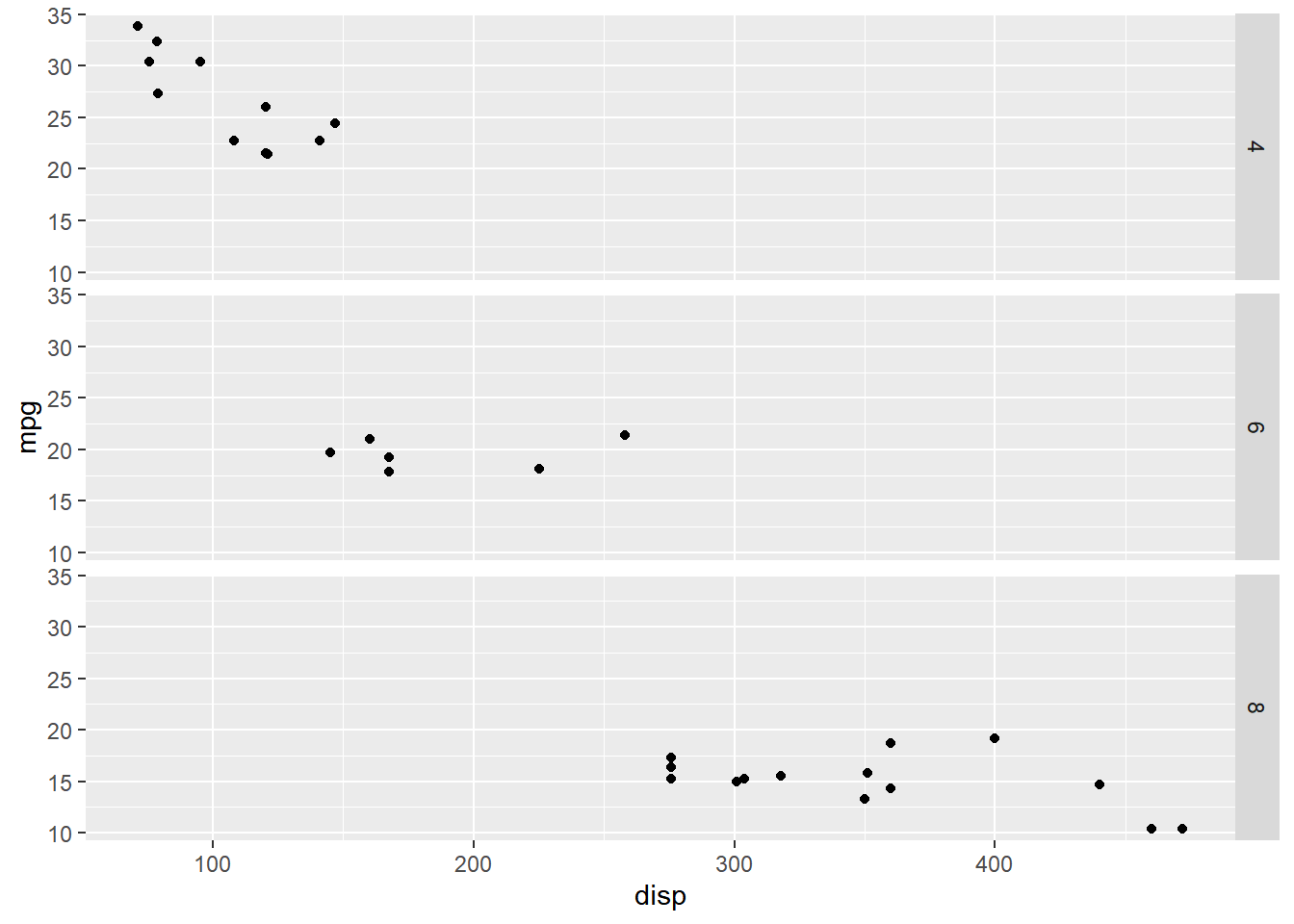Introduction This is the 19th post in the series Elegant Data Visualization with ggplot2. In the previous post, we learnt to modify the title, label and bar of a legend. In this post, we will learn about faceting i.e. combining plots. Libraries, Code & Data We will use the following libraries in this post: readr ggplot2 All the data sets used in this post can be found here...

ggplot2: Legend – Part 6

April 12, 2018
By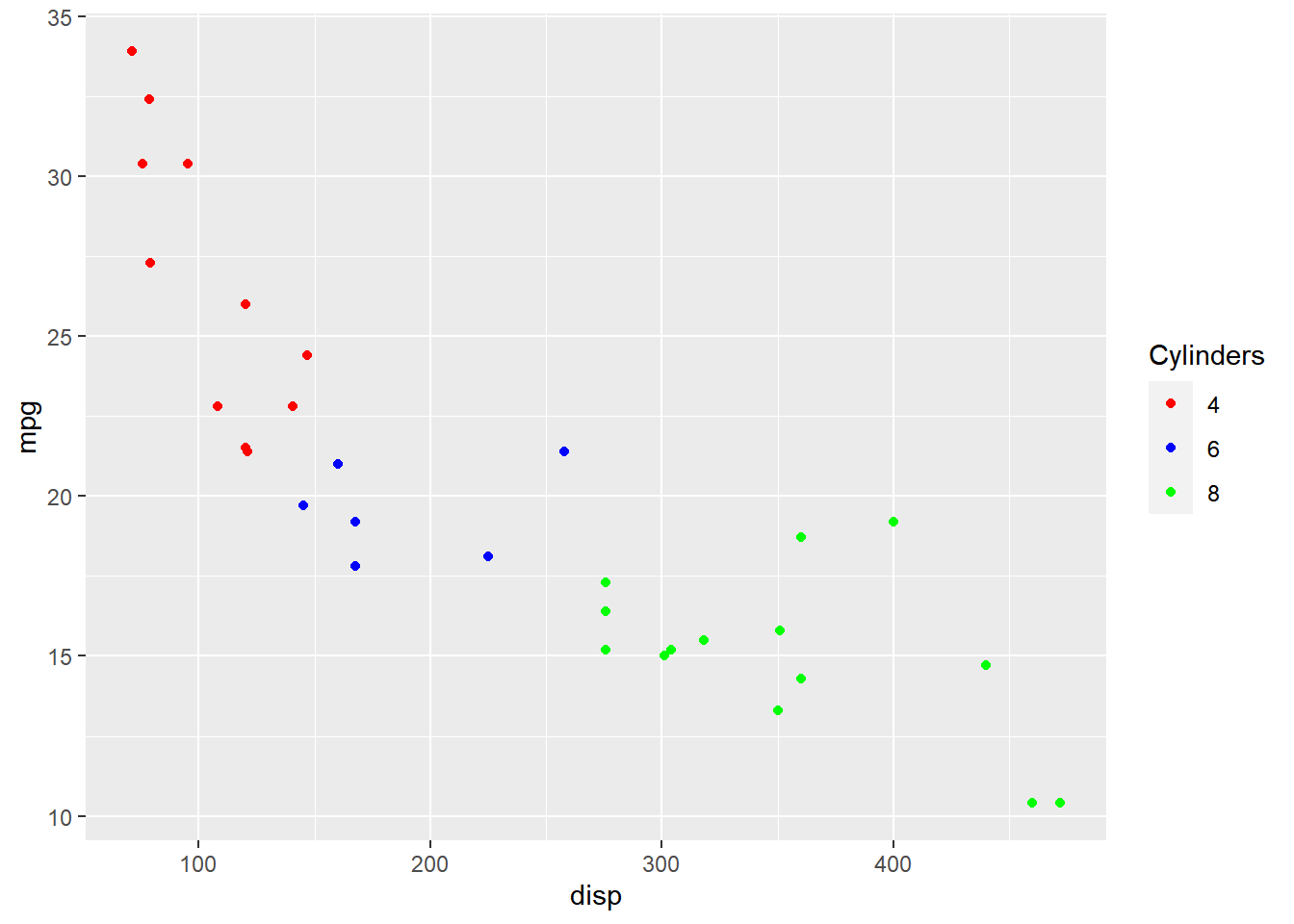Introduction This is the 18th post in the series Elegant Data Visualization with ggplot2. In the previous post, we learnt how to modify the legend of plot when alpha is mapped to a categorical variable. In this post, we will learn to modify legend title label and bar So far, we have learnt to modify the components of a legend using scale_* family of functions. Now, we...

ggplot2: Legend – Part 5

March 31, 2018
By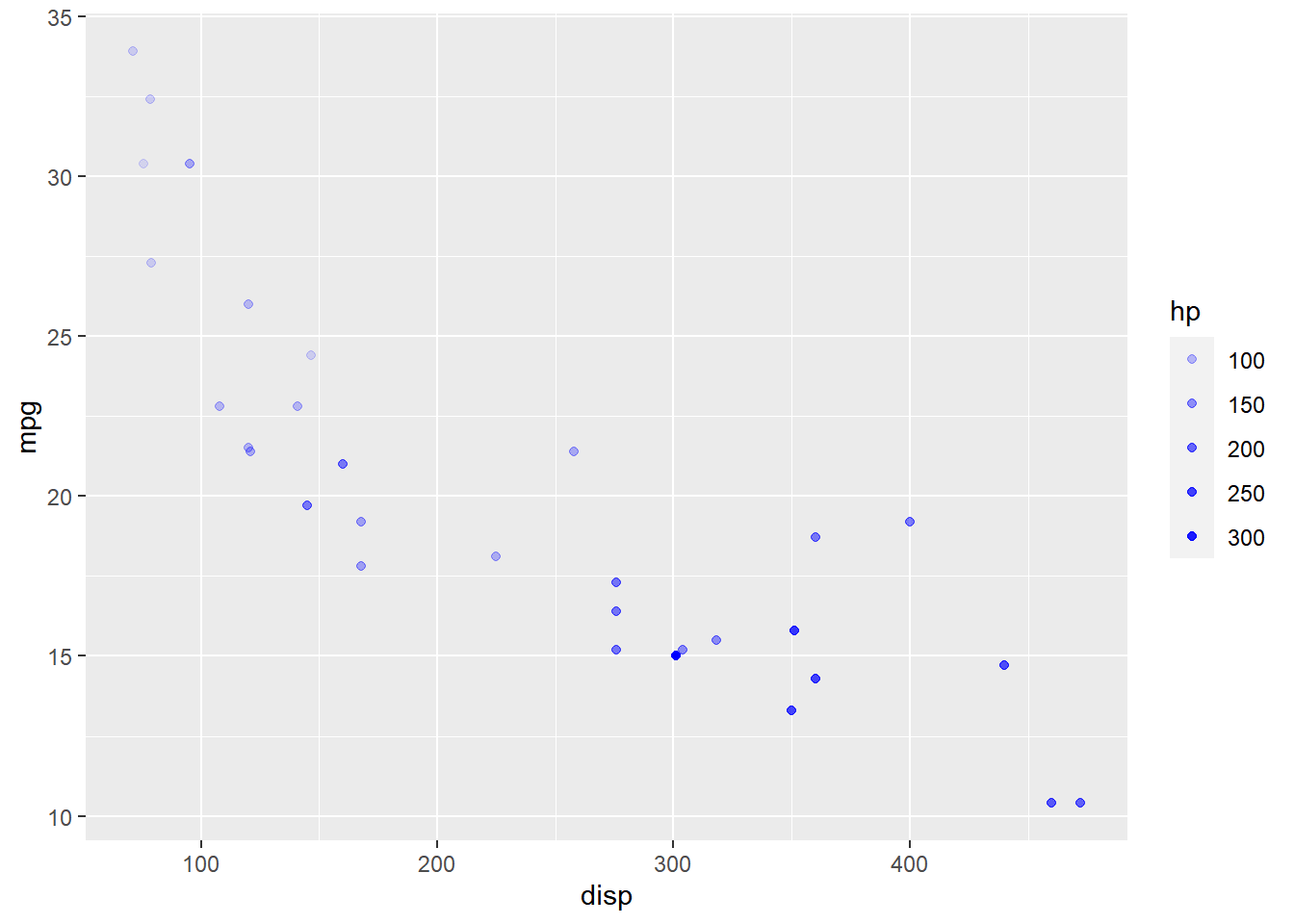Introduction This is the 17th post in the series Elegant Data Visualization with ggplot2. In the previous post, we learnt how to modify the legend of plot when size is mapped to continuous variable. In this post, we will learn to modify the following using scale_alpha_continuous() when alpha or transparency is mapped to variables: title breaks limits range labels values Libraries, Code & Data We will use the following libraries in this...

ggplot2: Legend – Part 4

March 19, 2018
By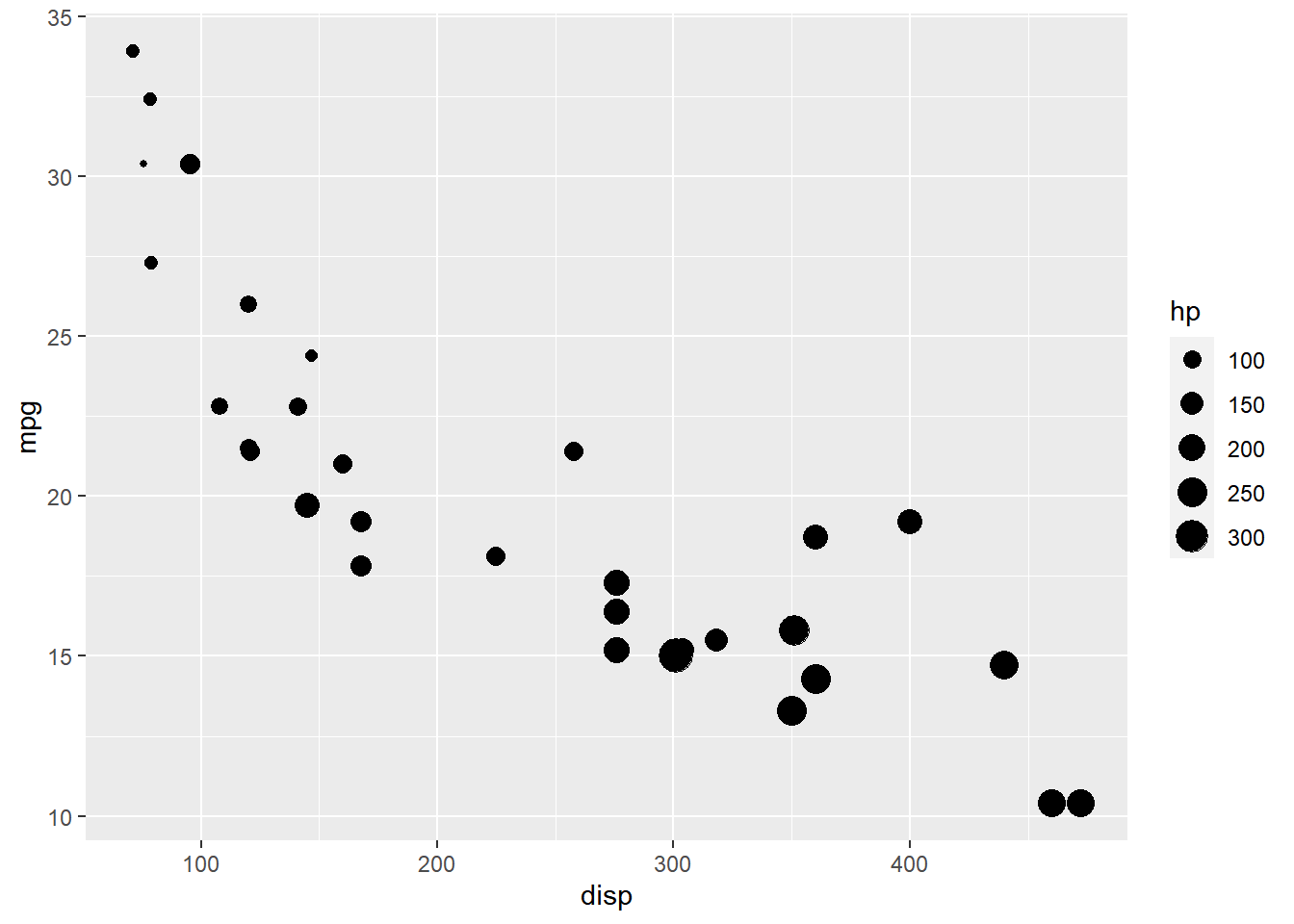Introduction This is the 16th post in the series Elegant Data Visualization with ggplot2. In the previous post, we learnt how to modify the legend of plot when shape is mapped to categorical variables. In this post, we will learn to modify the following using scale_size_continuous when size aesthetic is mapped to variables: title breaks limits range labels values Libraries, Code & Data We will use the following libraries in this post: readr ggplot2 All...

ggplot2: Legend – Part 3

March 7, 2018
By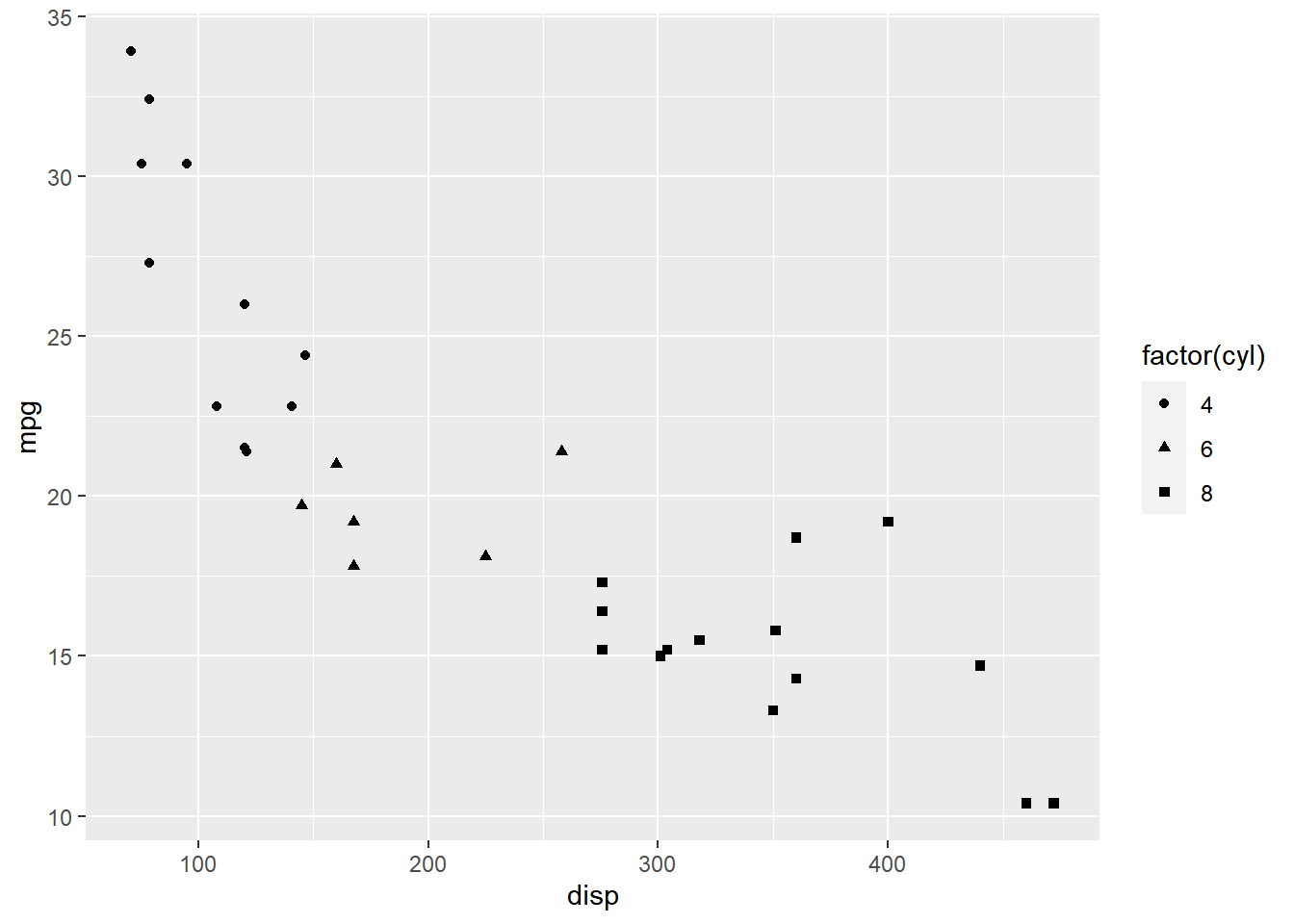Introduction This is the 15th post in the series Elegant Data Visualization with ggplot2. In the previous post, we learnt how to modify the legend of plots when aesthetics are mapped to variables..In this post, we will learn to modify the following using scale_shape_manual when shape is mapped to categorical variables: title breaks limits labels values Libraries, Code & Data We will use the following libraries in this post: readr ggplot2 All the data...

ggplot2: Legend – Part 2

February 23, 2018
ByIntroduction This is the 14th post in the series Elegant Data Visualization with ggplot2. In the previous post, we learnt how to modify the legend of plots when aesthetics are mapped to variables. In this post, we will continue to explore different ways to modify/customize the legend of plots. Specifically, we will learn to modify the following using scale_fill_manual() when fill is mapped to categorical...

ggplot2: Legend – Part 1

February 11, 2018
By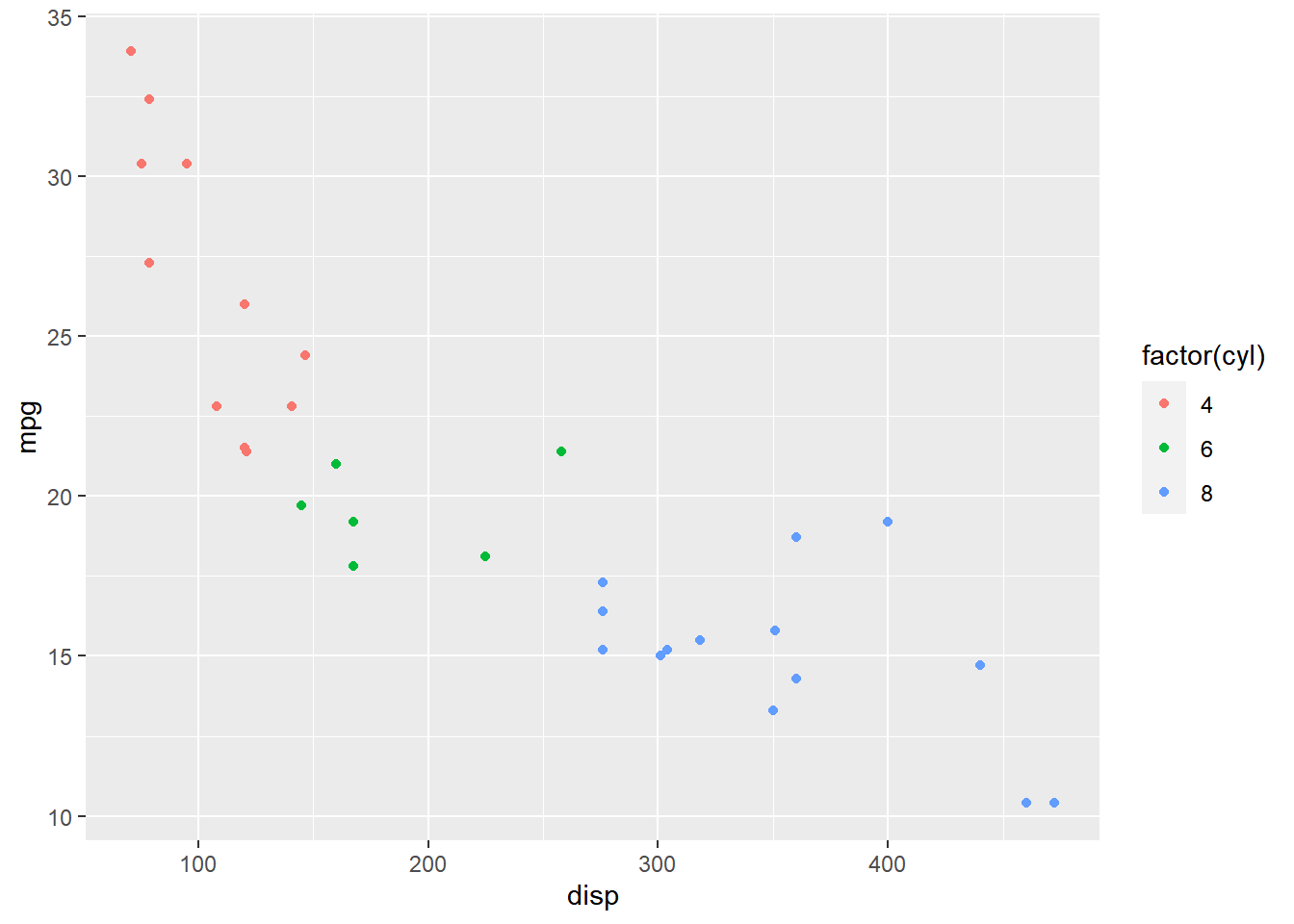Introduction This is the 13th post in the series Elegant Data Visualization with ggplot2. In the previos post, we learnt how to modify the axis of plots. In this post, we will focus on modifying the appearance of legend of plots when the aesthetics are mapped to variables. Specifically, we will learn to modify the following when color is mapped to categorical variables: title breaks limits labels values Libraries, Code...

ggplot2: Guides – Axes

January 30, 2018
By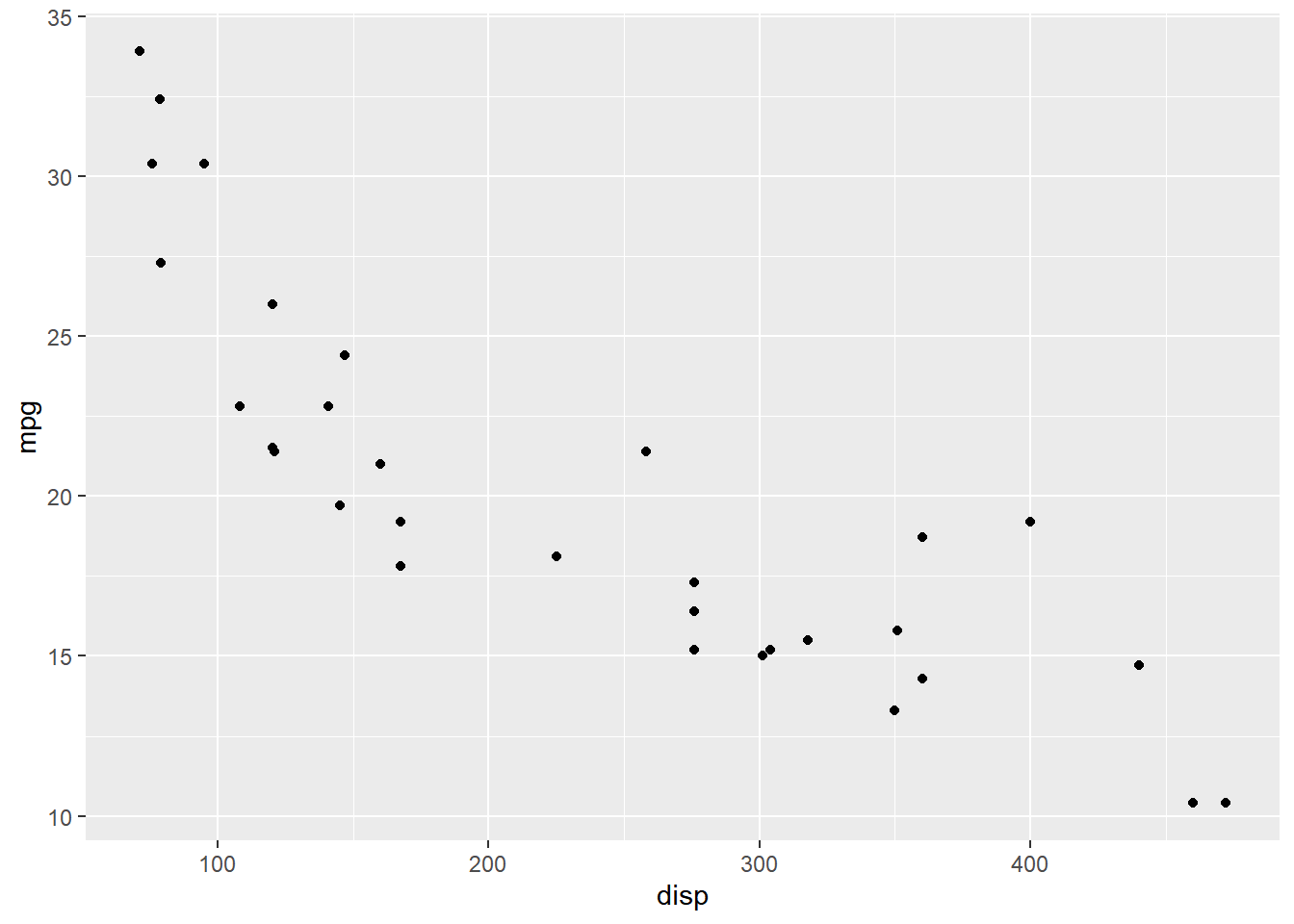Introduction This is the twelfth post in the series Elegant Data Visualization with ggplot2. In the previous post, we learnt to build histograms. Now that we have learnt to build different plots, let us look at different ways to modify the axis. Along the way, we will also explore the scale_*() family of functions. Modify X and Y axis title labels limits breaks position In this module, we will learn...

ggplot2: Histogram

January 18, 2018
By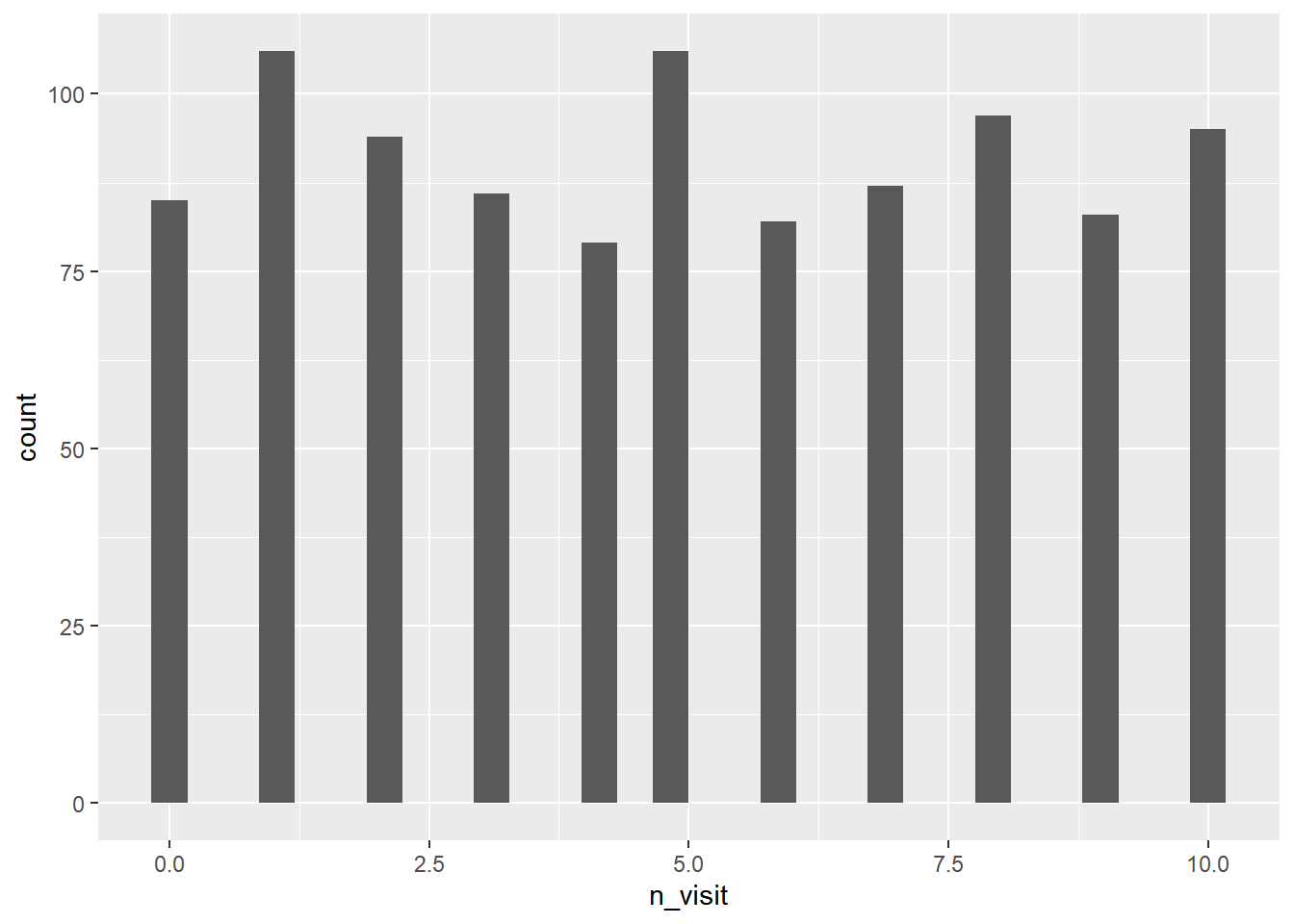Introduction This is the eleventh post in the series Elegant Data Visualization with ggplot2. In the previous post, we learnt to build box plots. In this post, we will learn to build histogram specify bins modify color fill alpha bin width line type line size map aesthetics to variables A histogram is a plot that can be used to examine the shape and spread of continuous data. It looks very similar to a...